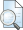## ANALISIS PRODUKSI BAWANG MERAH (Allium Ascalonicum) DI KECAMATAN REJOSO KABUPATEN NGANJUK

Anam, Mohamad Khoirul (2010) ANALISIS PRODUKSI BAWANG MERAH (Allium Ascalonicum) DI KECAMATAN REJOSO KABUPATEN NGANJUK. Other thesis, University of Muhammadiyah Malang.Preview
Text
ANALISIS_PRODUKSI_BAWANG_MERAH.pdf

## Abstract

The purpose of this research is to determine the effect of production cost of onion production and farmers to know how big influence of production costs on the yield of onion production. In this study, the hypothesis that taken by the researchers was that raw material costs and labor influence the production of red onion. The analysis tools to determine how far the influence of the cost of materials and labor on the yield of onion production using analysis of the Cobb­Douglas production function, using the formula Y = a x_1^b1 x_2^b2 x_3^b3… x_n^bn e. Further analysis of the forms of production function expressed in logarithmic form as follows: Log Y = Log a + b_1 Log x_1+ b_2 Log x_2 + b_3 Log x_3+ e. Result of calculation with the analytical tools Cobb­Douglas production function is Log Y = 2921516 + 3,996 X_1 ­ 2,284 X_2. From the analysis results proved that the cost of raw materials and labor influence the production of red onion. This is indicated by the overall significance level with a coefficient of determination (R^2) of 0,965 means that the two independent variables (X) effect on thedependent variable (Y) equal to 96,5% while the rest equal to 3,5% influenced by other factors. Based on the above conclusions, the authors could suggest that should the factors of production are already in a state such as the optimal raw material and labor usage is maintained in order to obtain optimal results.

Item Type: Thesis (Other) H Social Sciences > HB Economic Theory Faculty of Economics and Business > Department of Development Economics (60201) Rayi Tegar Pamungkas 03 Apr 2012 03:22 03 Apr 2012 03:22 http://eprints.umm.ac.id/id/eprint/1704View Item